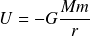# Gravitational energy

Gravitational energy characterizes the strength of a gravitational field. The formula quantifies the gravitational field in terms of its influence on the kinetic energy of a second body.

Gravitational energy potential is determined by the mass density operator acting on the maximum force potential. The formula also uses the rest mass operator to quantify the mass of a second body in relation to the Planck mass. The elementary formula stated in operators and potentials is

## Historical formula

The elementary formula can be derived from the historical formula for gravitational force which uses the gravitational constant. The historical formcan be re-stated in elementary form by replacing the gravitational constant with natural units of length, mass, and time. The formulacan then be grouped into operators and potentialsInserting a hidden quantity of Planck mass in the numerator and denominator states the formula in terms of two operators acting on the maximum Planck energy potential.The following illustration shows how the historical formula transforms into the natural formula with operators and potential.

Gravitational force: conversion from historical to natural formula

Gravitational energy